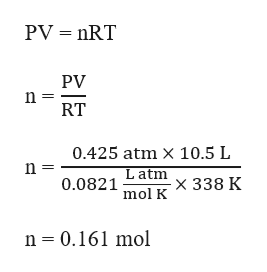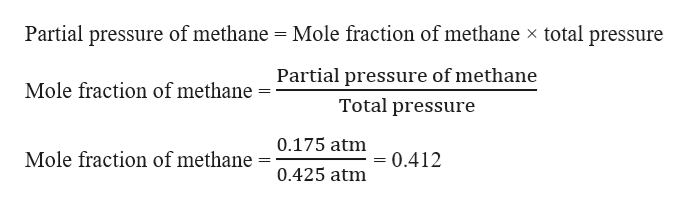# In a mixture of two gases, the partial pressure of methane is 0.175atm and the partial pressure of oxygen is 0.250atm.If the gases occupy a volume of 10.5 liters at 65 degrees Celsius, what is the number of moles of methane in the mixture?

Question
13 views

In a mixture of two gases, the partial pressure of methane is 0.175atm and the partial pressure of oxygen is 0.250atm.

If the gases occupy a volume of 10.5 liters at 65 degrees Celsius, what is the number of moles of methane in the mixture?

check_circle

star
star
star
star
star
1 Rating
Step 1

Given,

Partial pressure of methane = 0.175 atm

Partial pressure of oxygen = 0.250 atm

Volume, V = 10.5 L

Temperature, T = 65 oC = (65 + 273) K = 338 K

Step 2

Total pressure, P = 0.175 atm + 0.250 atm = 0.425 atm

The total number of moles (n) can be calculated using the ideal gas equation as:help_outlineImage TranscriptionclosePV nRT PV n = RT 0.425 atm X 10.5 L n = Latm 0.0821 x 338 K mol K n 0.161 mol fullscreen
Step 3

Mole fraction of methane ca...help_outlineImage TranscriptionclosePartial pressure of methane Mole fraction of methane x total pressure Partial pressure of methane Mole fraction of methane Total pressure 0.175 atm Mole fraction of methane =0.412 0.425 atm fullscreen

### Want to see the full answer?

See Solution

#### Want to see this answer and more?

Solutions are written by subject experts who are available 24/7. Questions are typically answered within 1 hour.*

See Solution
*Response times may vary by subject and question.
Tagged in

### Ideal gases